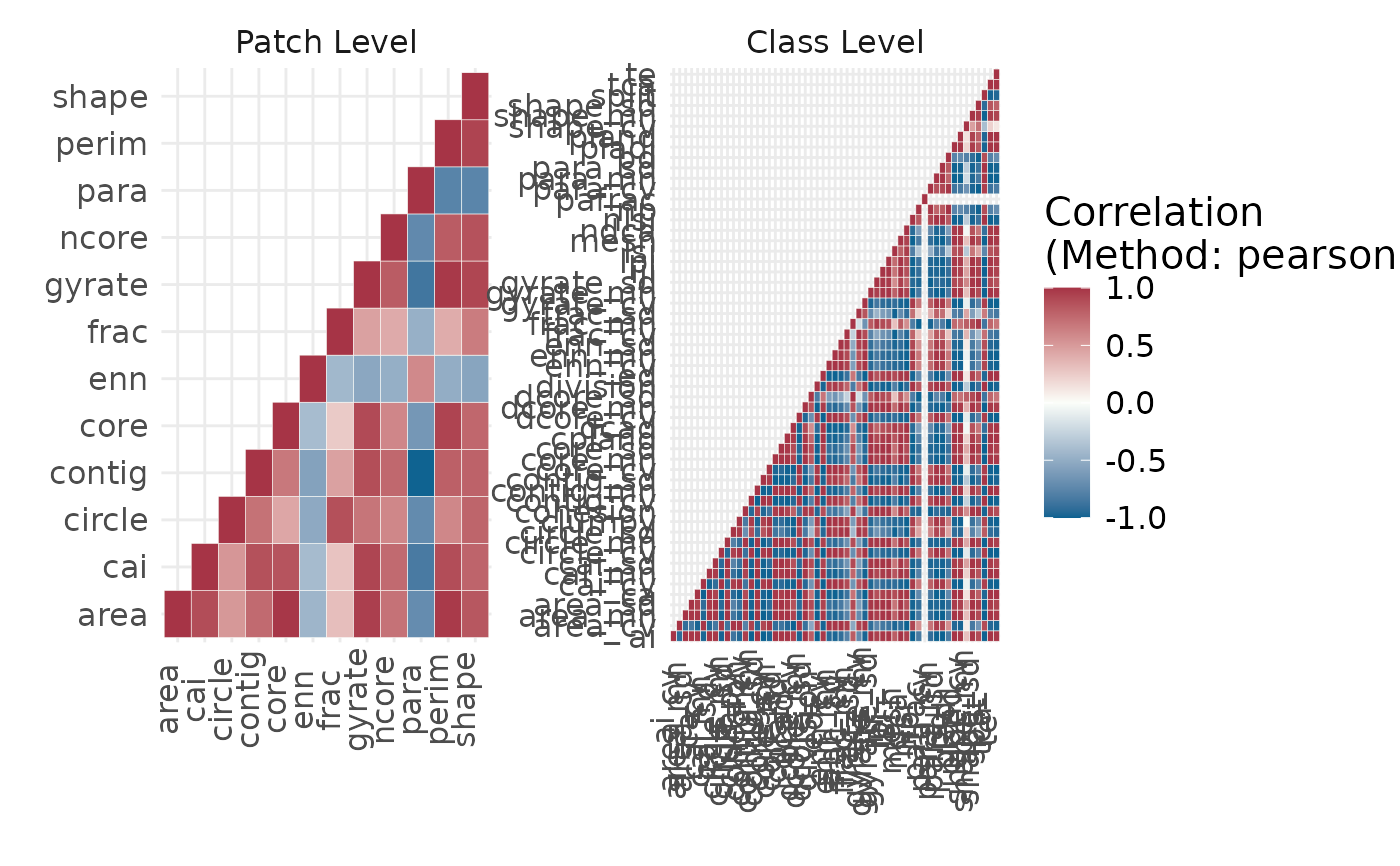Show correlation

## Usage

show_correlation(
data,
method = "pearson",
diag = TRUE,
labels = FALSE,
vjust = 0,
text_size = 15
)

## Arguments

data

Tibble with results of as returned by the landscapemetrics package.

method

Type of correlation. See link{cor} for details.

diag

If FALSE, values on the diagonal will be NA and not plotted.

labels

If TRUE, the correlation value will be added as text.

vjust

Will be passed on to ggplot2 as vertical justification of x-axis text.

text_size

Text size of the plot.

ggplot

## Details

The functions calculates the correlation between all metrics. In order to calculate correlations, for the landscape level more than one landscape needs to be present. All input must be structured as returned by the landscapemetrics package.

## Examples

landscape <- terra::rast(landscapemetrics::landscape)

metrics <- calculate_lsm(landscape, what = c("patch", "class"))
#> Warning: Please use 'check_landscape()' to ensure the input data is valid.
#> Warning: Class 1: PAFRAC = NA for class with < 10 patches
#> Warning: Class 3: PAFRAC = NA for class with < 10 patches
show_correlation(data = metrics, method = "pearson")if (FALSE) {
metrics <- calculate_lsm(landscape, what = c("patch", "class"))#'
correlations <- calculate_correlation(metrics)
show_correlation(data = correlations, method = "pearson")
}